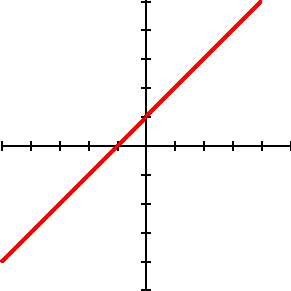# graph of an equation or inequality

Graph of an Equation or Inequality

The picture obtained by plotting all the points of an equation or inequality.

If there is only one variable, the graph is on a number line. If there are two variables, the graph is on the coordinate plane. If there are three variables, the graph is in three-dimensional coordinates. In general, for n variables, the graph is in n dimensions.

For example, the graph of the equation y = x + 1 given below is obtained by plotting all the points with a y-coordinate that is 1 larger than the x-coordinate.